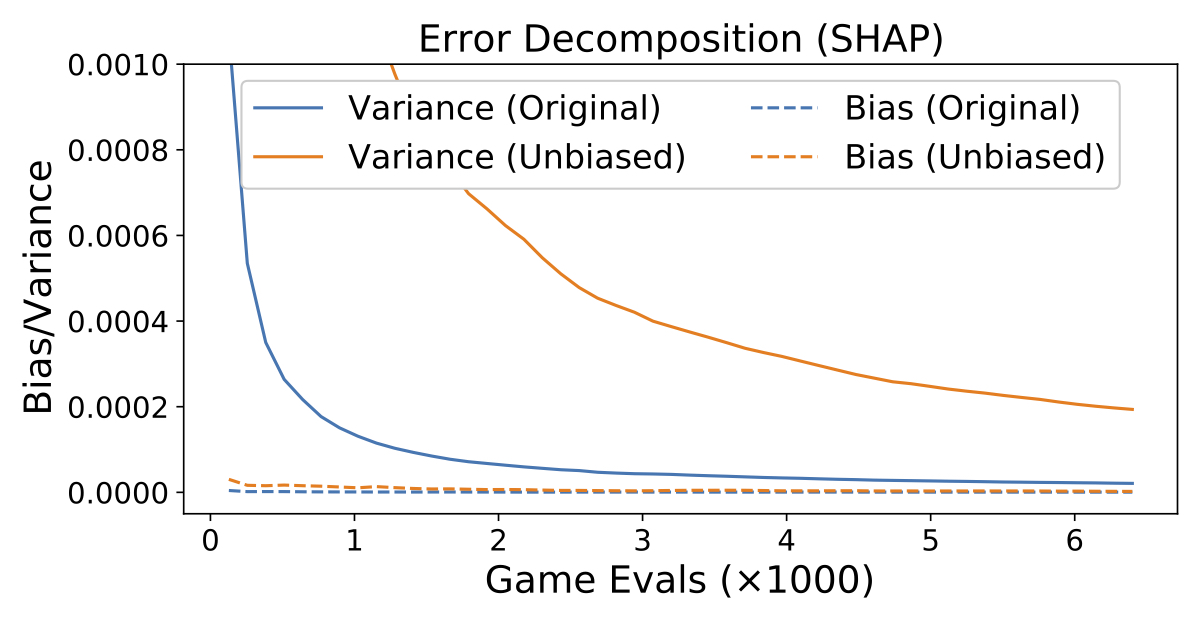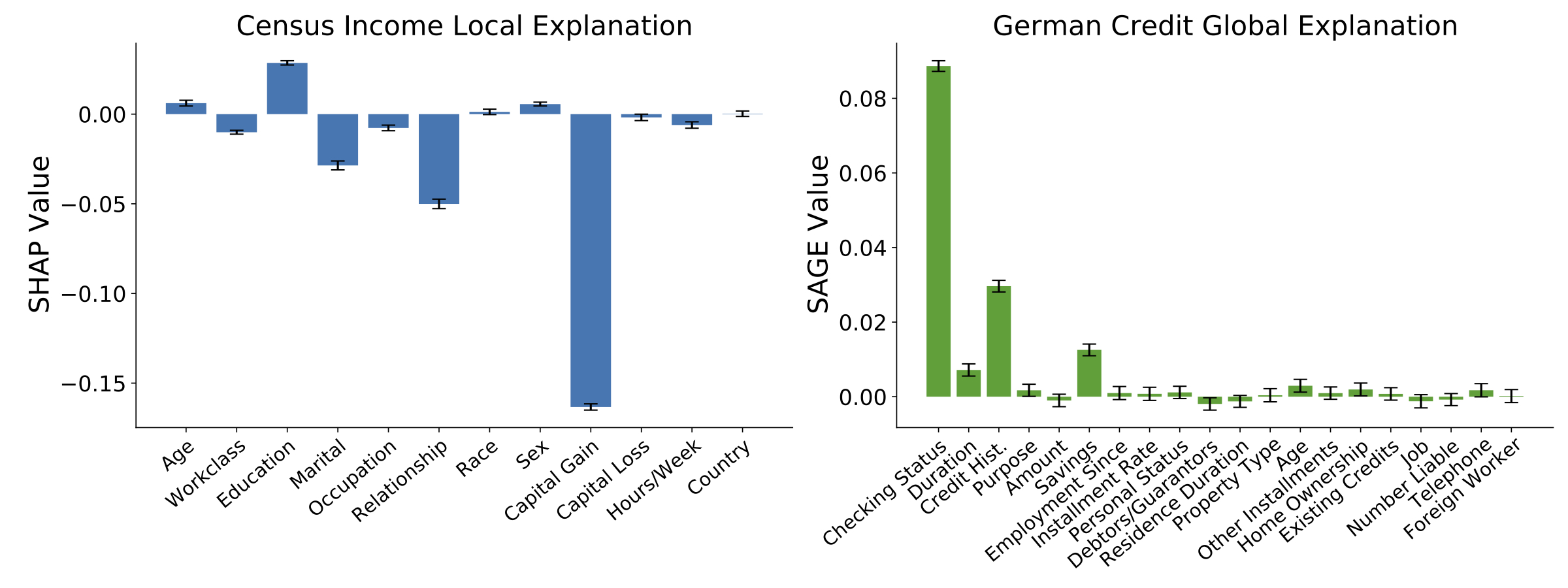# Understanding and improving KernelSHAP

December 8, 2020

KernelSHAP is a popular implementation of SHAP, but it has several shortcomings that make it difficult to use in practice. In our recent AISTATS paper , we revisited the regression-based approach for estimating Shapley values to better understand and ultimately improve upon KernelSHAP.

Our goal was making Shapley value estimation more practical, and we did so by developing techniques for:

1. Providing uncertainty estimates
2. Detecting convergence automatically
3. Accelerating convergence
4. Generalizing KernelSHAP to other Shapley value-based explanation methods

This post describes our paper at a high level, while avoiding some of the mathematical details.

## Background

First, let's review the differences between KernelSHAP, SHAP and Shapley values. (If you're unfamiliar with cooperative games or Shapley values, check out this post.)

Shapley values are a credit allocation scheme for cooperative games (i.e., set functions) , and, given a game $v$ that accepts coalitions/subsets $S \subseteq D \equiv \{1, 2, \ldots d\}$, the Shapley values for each player $i \in D$ are defined as follows:

Next, SHAP is an interpretability method that explains individual predictions by assigning attribution scores to each feature $x_i$ using Shapley values . Given a model $f$ and an input $x$, SHAP is based on a cooperative game $v_x$ defined as follows (when using the conditional distribution for missing features):

SHAP values are feature attribution scores given by the Shapley values $\phi_i(v_x)$. Because of how Shapley values are derived, SHAP values represent how much each feature moves the prediction $f(x)$ up or down relative to the base-rate prediction $\mathbb{E}[f(X)]$.

Finally, KernelSHAP is a method for approximating SHAP values . Shapley values are hard to calculate, so SHAP is only useful in theory without an approximation technique. KernelSHAP is the main practical implementation of SHAP, and since the features' conditional distributions are often unknown, KernelSHAP is typically run with a slightly different cooperative game that uses the marginal distribution for missing features:

Table 1 summarizes these three related ideas.

Table 1: Shapley values, SHAP and KernelSHAP.
What it means Introduced by
Shapley values Credit allocation method for cooperative games 
SHAP Model explanation method (Shapley values of
a particular cooperative game)

KernelSHAP Approximation approach for SHAP values 

KernelSHAP has become the preferred method for model-agnostic SHAP value calculation , but recent work has exposed certain weaknesses in KernelSHAP. For example, Merrick and Taly  raised questions about whether KernelSHAP is an unbiased estimator (or a statistical estimator in any sense), and the SAGE paper  showed that sampling-based approximations permit convergence detection and uncertainty estimation, whereas KernelSHAP offers neither. These are some of the issues we address in our paper, "Improving KernelSHAP: Practical Shapley Value Estimation via Linear Regression" .

## KernelSHAP

KernelSHAP approximates Shapley values by solving a linear regression problem. Thanks to the original SHAP paper , we know that the Shapley values for a cooperative game $v$ are the solutions to the weighted least squares problem

where the weighting function $\mu_{\text{Sh}}$ is given by:

Solving weighted least squares problems is usually easy, but this one is difficult because it has $2^d$ input-output pairs (or data points) $\big(S, v(S)\big)$, each of which may be costly to calculate. KernelSHAP therefore uses a dataset sampling approach (described in detail in our paper) that involves solving a constrained least squares problem with a manageable number of data points. I'll omit the equations here, but this problem can be solved without much difficulty using its KKT conditions.

This describes KernelSHAP at a high level. It's worth mentioning, however, that KernelSHAP need not be used in the context of SHAP: the equations above are not specific to the game used by SHAP ($v_x$), so this regression-based approach can be applied to any cooperative game. (We will continue to use the name KernelSHAP, but we now mean the regression-based approach to approximating Shapley values.)

KernelSHAP is simple to implement, but its properties were unknown until now. As prior work pointed out, it's not obvious in what sense the dataset sampling approach even represents a statistical estimator. In our paper, we improved our understanding of KernelSHAP by discovering the following properties:

• KernelSHAP is a consistent Shapley value estimator.
• KernelSHAP has negligible bias.
• KernelSHAP has significantly lower variance than our newly proposed unbiased KernelSHAP estimator. The new estimator has the advantage of being provably unbiased, but the original approach appears to make a more favorable bias-variance trade-off (see Figure 1).
• KernelSHAP's variance evolves at a rate of $\mathcal{O}(\frac{1}{n})$ with the number of sampled data points, identical to the unbiased KernelSHAP estimator.Figure 1: Error decomposition (bias-variance trade-off) for original and unbiased KernelSHAP on a single census income prediction.

Given these properties, the original KernelSHAP approach seems preferable to our provably unbiased estimator (described in the paper). The dataset sampling approach is not provably unbiased, but because it converges faster and its variance is empirically near zero, users should prefer this approach in practice.

Beyond improving our understanding of KernelSHAP, knowing that its variance evolves at a rate of $\mathcal{O}(\frac{1}{n})$ allows us to modify KernelSHAP with an online variance approximation. Over the course of estimation with $n$ samples, we produce multiple independent estimates with $m$ samples (for $m << n$) and use these to approximate the variance for the final results. The variance approximation can then be used to provide confidence intervals, and to automatically determine the required number of samples (rather than using an arbitrary number or the default argument). Figure 2 shows what explanations look like when run with these new modifications.Figure 2: Shapley value-based explanations with 95% uncertainty estimates. Left: SHAP values for a single prediction with the census income dataset. Right: SAGE values for the German credit dataset.

Finally, our paper presented two more innovations for KernelSHAP:

1. We presented a paired sampling approach that accelerates KernelSHAP's convergence. The technique is simple: each sampled subset $S \subseteq D$ is paired with the complement subset $D \setminus S$. In most cases, this approach significantly reduces the variance for the original KernelSHAP, enabling it to converge roughly $10\times$ faster on the four datasets examined in our experiments.
2. We adapted KernelSHAP to the setting of stochastic cooperative games to develop fast estimators for two global explanation methods, SAGE  and Shapley Effects . Stochastic cooperative games have not previously been used in the model explanation literature, but we find that these tools provide a helpful way of re-framing these methods and deriving fast estimators. The approximation approach we introduce is significantly faster than baselines in our experiments.

With these new insights and tools, we hope to provide users with a more practical approach to Shapley value estimation via linear regression. Shapley values still aren't cheap to calculate relative to certain other methods, but you can now calculate them faster and be confident that you're using the right number of samples.

## Conclusion

Shapley values are used by several promising model explanation methods (SHAP, SAGE, Shapley Effects, etc.), but fast approximations are required to use these methods in practice. Our paper develops a practical approach using a linear regression-based estimator, providing features like uncertainty estimatation and automatic convergence detection. If you want to try out our approach, our implementation is online and you can apply it with model explanation methods (such as SHAP) or with arbitrary cooperative games.

## References

1. Ian Covert, Su-In Lee. "Improving KernelSHAP: Practical Shapley Value Estimation via Linear Regression." Artificial Intelligence and Statistics, 2021.
2. Lloyd Shapley. "A Value for n-Person Games." Contributions to the Theory of Games, 1953.
3. Scott Lundberg, Su-In Lee. "A Unified Approach to Interpreting Model Predictions." Neural Information Processing Systems, 2017.
4. Luke Merrick, Ankur Taly. "The Explanation Game: Explaining Machine Learning Models Using Shapley Values." CD-Make 2020.
5. Ian Covert, Scott Lundberg, Su-In Lee. "Understanding Global Feature Contributions Through Additive Importance Measures." Neural Information Processing Systems, 2020.
6. Art Owen. "Sobol' Indices and Shapley value." SIAM 2014.